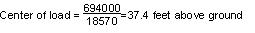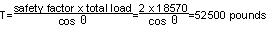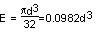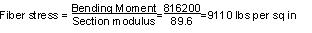Previous Rule Change Main Page Resolution Decision Main Page Change List for this Rule Search Decision Number 14-02-015 Decision Number 14-02-015 Next Rule Change

Original Version
Appendix F - Part 2 - #3

3. Pole (See Page F–22)

Rule 44 provides that poles supporting unbalanced longitudinal loads in Grade “A” construction shall have a safety factor of 4 against such loads. Rule 47.3 specifies that guys used to support unbalanced longitudinal loads shall have a safety factor of 2 for all grades of construction (Where guys are used they must take the entire load with the designated safety factor, the pole being considered merely as a strut).

Using the values given above for tensions at maximum loading, the following moments due to dead ending the conductors are obtained:

 3 x 2,125 x 47.3 =301,500 pound–feet 4 x 1,360 x 38.3 =208,400 pound–feet 3 x 890 x 30.3 =80,900 pound–feet 3 x 1,360 x 25.3 =103,200 pound–feet Total Moments =694,000 pound–feet

The total deadend stress, using the tension values for maximum loading given above, will be:

 3 x 2,125 =6,380 pounds 4 x 1,360 =5,440 pounds 3 x 890 =2,670 pounds 3 x 1,360 =18,570 pounds Total Moments =694,000 pound–feetThe tension of a single guy with a lead to height ratio of 1 to 1 (assumed) and a safety factor of 2 would be:A stranded guy attached at the center of load could be used provided the allowable fiber stress of the pole is not exceeded. The stress due to guying at this point would be as follows:

The center of load (37.4’ above ground) would be

9.9 ft. (118.8”) below the top conductors (11 kV) and

0.9 ft. ( 10.8”) below the second crossarm (4 kV)

The fiber stress in the pole at the center of load due to the tension in the conductors above the center of load is computed as follows:

 Bending moment 3 x 2,125 x 118.8 = 757,400 pound–inches 4 x 1,360 x 10.8 = 58,800 pound–inches Total moment = 816,200 pound–inches

The section modulus of a solid circular section isThen, E = 0.0982 x (9.7)3 = 89.6” 3Since a pole in Grade “A” construction must have a safety factor of 4, the allowable value of fiber stress would be 5,600/4 = 1,400 pounds per square inch; therefore, the pole cannot be guyed by a single guy but can be guyed as illustrated on Page F–22.

Strikeout and Underline Version
Appendix F - Part 2 - #3

3. Pole (See Page F–22)

Rule 44 provides that poles supporting unbalanced longitudinal loads in Grade “A” construction shall have a safety factor of 4 against such loads. Rule 47.3 specifies that guys used to support unbalanced longitudinal loads shall have a safety factor of 2 for all grades of construction (Where guys are used they must take the entire load with the designated safety factor, the pole being considered merely as a strut).

Using the values given above for tensions at maximum loading, the following moments due to dead ending the conductors are obtained:

 3 x 2,125 x 47.3 =301,500 pound–feet 4 x 1,360 x 38.3 =208,400 pound–feet 3 x 890 x 30.3 =80,900 pound–feet 3 x 1,360 x 25.3 =103,200 pound–feet Total Moments =694,000 pound–feet

The total deadend stress, using the tension values for maximum loading given above, will be:

 3 x 2,125 =6,380 pounds 4 x 1,360 =5,440 pounds 3 x 890 =2,670 pounds 3 x 1,360 =18,570 pounds Total Moments =694,000 pound–feetThe tension of a single guy with a lead to height ratio of 1 to 1 (assumed) and a safety factor of 2 would be:A stranded guy attached at the center of load could be used provided the allowable fiber stress of the pole is not exceeded. The stress due to guying at this point would be as follows:

The center of load (37.4’ above ground) would be

9.9 ft. (118.8”) below the top conductors (11 kV) and

0.9 ft. ( 10.8”) below the second crossarm (4 kV)

The fiber stress in the pole at the center of load due to the tension in the conductors above the center of load is computed as follows:

 Bending moment 3 x 2,125 x 118.8 = 757,400 pound–inches 4 x 1,360 x 10.8 = 58,800 pound–inches Total moment = 816,200 pound–inches

The section modulus of a solid circular section isThen, E = 0.0982 x (9.7)3 = 89.6” 3Since a pole in Grade “A” construction must have a safety factor of 4, the allowable value of fiber stress would be 5,600/4 = 1,400 pounds per square inch; therefore, the pole cannot be guyed by a single guy but can be guyed as illustrated on Page F–22.

Final Version
Appendix F - Part 2 - #3

3. Pole (See Page F–22)

Rule 44 provides that poles supporting unbalanced longitudinal loads in Grade “A” construction shall have a safety factor of 4 against such loads. Rule 47 specifies that guys used to support unbalanced longitudinal loads shall have a safety factor of 2 for all grades of construction (Where guys are used they must take the entire load with the designated safety factor, the pole being considered merely as a strut).

Using the values given above for tensions at maximum loading, the following moments due to dead ending the conductors are obtained:

 3 x 2,125 x 47.3 =301,500 pound–feet 4 x 1,360 x 38.3 =208,400 pound–feet 3 x 890 x 30.3 =80,900 pound–feet 3 x 1,360 x 25.3 =103,200 pound–feet Total Moments =694,000 pound–feet

The total deadend stress, using the tension values for maximum loading given above, will be:

 3 x 2,125 =6,380 pounds 4 x 1,360 =5,440 pounds 3 x 890 =2,670 pounds 3 x 1,360 =18,570 pounds Total Moments =694,000 pound–feetThe tension of a single guy with a lead to height ratio of 1 to 1 (assumed) and a safety factor of 2 would be:A stranded guy attached at the center of load could be used provided the allowable fiber stress of the pole is not exceeded. The stress due to guying at this point would be as follows:

The center of load (37.4’ above ground) would be

9.9 ft. (118.8”) below the top conductors (11 kV) and

0.9 ft. ( 10.8”) below the second crossarm (4 kV)

The fiber stress in the pole at the center of load due to the tension in the conductors above the center of load is computed as follows:

 Bending moment 3 x 2,125 x 118.8 = 757,400 pound–inches 4 x 1,360 x 10.8 = 58,800 pound–inches Total moment = 816,200 pound–inches

The section modulus of a solid circular section isThen, E = 0.0982 x (9.7)3 = 89.6” 3Since a pole in Grade “A” construction must have a safety factor of 4, the allowable value of fiber stress would be 5,600/4 = 1,400 pounds per square inch; therefore, the pole cannot be guyed by a single guy but can be guyed as illustrated on Page F–22.